# Multiplication - Building Arrays: Grade 2

##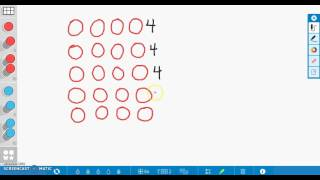By university of Houston mathematics education

The video describes a student strategy for multiplication by building an array. The video also includes repeated addition and skip counting.# [3.NBT.2-1.0] Fluent Add/Sub - Common Core Standard

##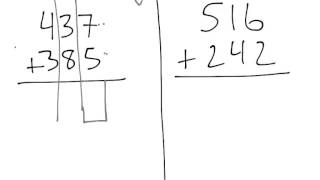By Freckle education

Fluently add within 1000 using strategies and algorithms based on place value, properties of operations, and/or the relationship between addition and subtraction.# [3.NBT.2-2.0] Fluent Add/Sub - Common Core Standard - Word Problem

##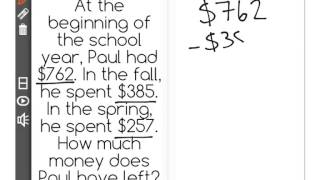By Freckle education

Fluently subtract within 1000 using strategies and algorithms based on place value, properties of operations, and/or the relationship between addition and subtraction.# 2.NBT.9

##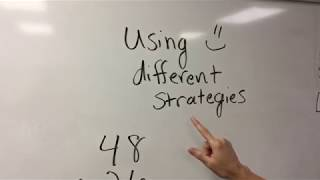By Teresa Plachecki

2nd grade math lesson on using different strategies to solve double digit addition problems.# 2.NBT.9

##By Teresa Plachecki

2nd grade math lesson on using different strategies to solve double digit addition problems.# [4.NF.3a-1.0] Intro to Fraction Addition - Common Core Standard - Word Problem

##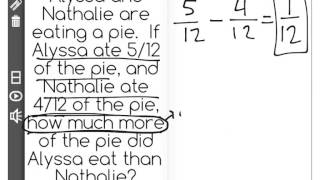By Freckle education

Understand a fraction a/b with a greater than 1 as a sum of fractions 1/b. Understand addition and subtraction of fractions as joining and separating parts referring to the same whole.# [4.NF.3a-1.0] Intro to Fraction Addition - Common Core Standard

##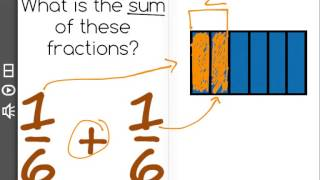By Freckle education

Understand a fraction a/b with a greater than 1 as a sum of fractions 1/b. Understand addition and subtraction of fractions as joining and separating parts referring to the same whole.##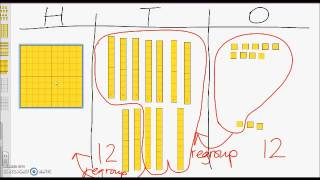By university of Houston mathematics education

The video describes how elementary students can solve an addition word problem regrouping tens and ones.# Vector Addition and Scalar Multiplication, Example 1

##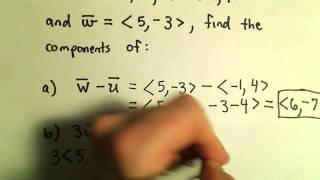By PatrickJMT

Vector Addition and Scalar Multiplication, Example 1. In this video, we look at vector addition and scalar multiplication algebraically using the component form of the vector. I do not graph the vectors in this video (but do in others).# Vector Addition and Scalar Multiplication, Example 2

##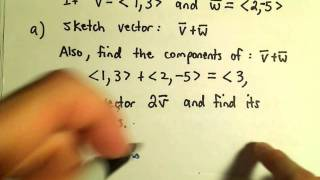By PatrickJMT

Vector Addition and Scalar Multiplication, Example 2. In this video I add two vectors in component form and also sketch the vectors to illustrate how to add vectors graphically (very useful stuff!).# Subtraction

##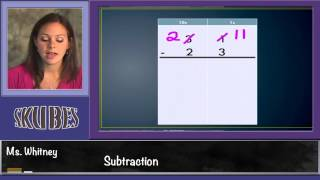By Skubes ed

Fluently add and subtract within 1000 using strategies and algorithms based on place value, properties of operations, and/or the relationship between addition and subtraction.##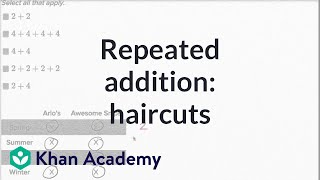By Khan Academy

Learn how to solve word problems by adding the same number many times.##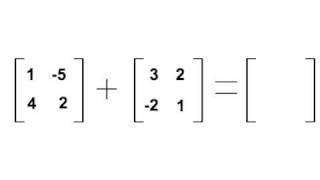By Joel Walkley# Addition formulae Sin(A+B), Cos(A+B), Tan (A+B): ExamSolutions

##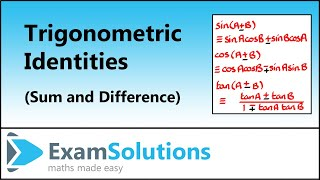By ExamSolutions

What are the addition formulae. To see the full index of tutorials go to ExamSolutions at http://www.examsolutions.co.uk# More on matrix addition and scalar multiplication | Linear Algebra | Khan Academy

##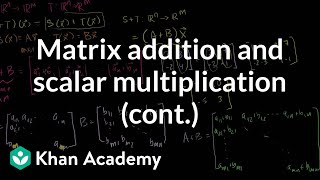By Khan Academy

More on Matrix Addition and Scalar Multiplication# More on matrix addition and scalar multiplication | Linear Algebra | Khan Academy

##By Khan Academy

More on Matrix Addition and Scalar Multiplication# Vector Addition and Scalar Multiplication, Example 2

##By PatrickJMT

Vector Addition and Scalar Multiplication, Example 2. In this video I add two vectors in component form and also sketch the vectors to illustrate how to add vectors graphically (very useful stuff!).# Associative Property of Addition and Multiplication

##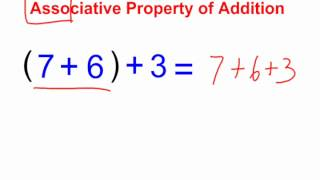By SmithMathAcademy

Associative Property of Addition and Multiplication# Addition Math Facts to 20

##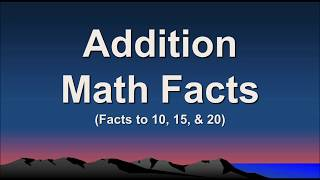By Anna Navarre

This is an addition math facts video with sums to 20.# [4.NF.3d-1.0] Word Problems: Addition - Common Core Standard

##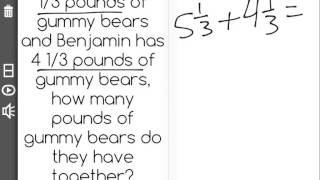By Freckle education

Solve word problems involving addition and subtraction of fractions referring to the same whole and having like denominators, e.g., by using visual fractions models and equations to represent to problem.